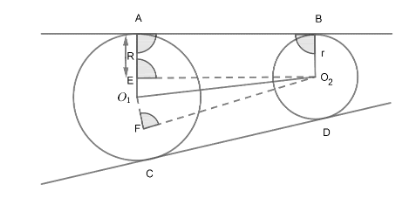QuestionAnswers

# Draw two direct common tangents to two circles of radii 4cm and 2cm whose centres are 8cm apart. Measure the length of the tangents.Hint:We will be using the concept of circle tangents to solve the problem. Also, some concepts of geometry and trigonometry will be used. Remember the tangent drawn to the circle is perpendicular to the radius at the point of contact.Now, we have to find the length of direct tangents to the circles. We have been given that the radius of two circles are 4cm and 2cm respectively.
We will first draw a diagram to clear the situation.

We have been given that radius of circles are R = 4cm & r = 2cm………(1)
Also, the distance between the two centres is $8cm={{O}_{1}}{{O}_{2}}..........\left( 2 \right)$
Now, to find the length of AB, we will draw perpendicular from ${{O}_{2}}$ to AO, as shown in the figure.
Now, in quadrilateral $AO,{{O}_{2}}B$ all the angles are $90{}^\circ$ . Since, tangent is perpendicular to radius and O,F is perpendicular to AO. Therefore, $AO,{{O}_{2}}B$ is a rectangle.
Now, In $\Delta O,{{O}_{2}}E$ , we know that $\angle {{O}_{2}}E{{O}_{1}}=90{}^\circ$ .
Therefore, by applying Pythagoras theorem,
\begin{align} & {{O}_{1}}{{O}_{2}}^{2}=E{{O}_{1}}^{2}+E{{O}_{2}}^{2} \\ & {{O}_{1}}{{O}_{2}}^{2}={{\left( R-r \right)}^{2}}+E{{O}_{2}}^{2} \\ \end{align}
Now using (1) and (2),
\begin{align} & {{\left( 8 \right)}^{2}}={{\left( 4-2 \right)}^{2}}+{{\left( E{{O}_{2}} \right)}^{2}} \\ & 64=4+{{\left( E{{O}_{2}} \right)}^{2}} \\ & E{{O}_{2}}=\sqrt{64-4} \\ & E{{O}_{2}}=\sqrt{60} \\ & =2\sqrt{15}cm \\ \end{align}
Now, $AB=E{{O}_{2}}$ . Since, opposite sides of the rectangle are equal. So, $AB=2\sqrt{15}cm$ .
Similarly, the length of the tangent CD will be equal to that of AB as the exact same process is required to find it and since the constants remain the same we will get the same answer therefore, the length of the direct common tangents is $2\sqrt{15}cm$ .

Note: These types of questions are usually tricky to do the first time but if one remembers the concepts of geometry it can be solved easily. Also we have to construct a perpendicular on our own to solve the question. This is a very important concept. properties of tangent drawn to the circle and pythagoras theorem is important here to get the solution.
View Notes
CBSE Class 8 Maths Chapter 13 - Direct and Inverse Proportions FormulasCBSE Class 10 Maths Chapter 10 - Circles FormulaCommon ColdCBSE Class 10 Maths Chapter 3 - Pair of Linear Equations in Two Variables FormulaCommon Diseases in Humans - MalariaPair of Linear Equation in Two VariablesCross Vector Product of Two VectorsTo Determine the Mass of Two Different Objects Using a Beam BalanceConsumer Equilibrium in Case of Two CommodityCBSE Class 10 Maths Chapter 8 - Introduction to Trigonometry FormulaCBSE Class 10 Maths Question Paper 2017CBSE Class 10 Maths Question Paper 2020Maths Question Paper for CBSE Class 10 - 2011Maths Question Paper for CBSE Class 10 - 2008Maths Question Paper for CBSE Class 10 - 2012Maths Question Paper for CBSE Class 10 - 2009Maths Question Paper for CBSE Class 10 (2016)Maths Question Paper for CBSE Class 10 - 2010Maths Question Paper for CBSE Class 10 - 2007CBSE Class 10 Maths Question Paper 2018 - Free PDFNCERT Solutions for Class 8 Maths Chapter 13 Direct and Inverse ProportionsNCERT Solutions for Class 10 Maths Chapter 12 Areas Related to CirclesRD Sharma Solutions for Class 10 Maths Chapter 15 - Areas Related to CirclesNCERT Solutions for Class 10 Maths Chapter 10 CirclesRD Sharma Solutions for Class 10 Maths Chapter 10 - CirclesNCERT Solutions for Class 9 Maths Chapter 10 CirclesRS Aggarwal Class 10 Solutions - CirclesRD Sharma Class 8 Maths Solutions Chapter 10 - Direct and Inverse variationsNCERT Solutions for Class 10 Maths Chapter 3 Pair of Linear Equations in Two VariablesRD Sharma Solutions for Class 10 Maths Chapter 3 - Pair of Linear Equations in Two Variables